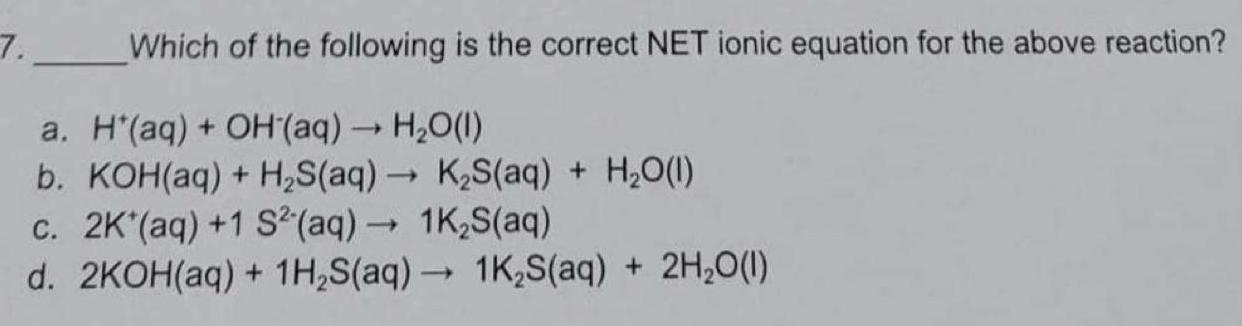Question:

# Which of the following is the correct NET ionic equation for

Last updated: 8/8/2022Which of the following is the correct NET ionic equation for the above reaction? a. H+(aq) + OH(aq) → H₂O(l) b. KOH(aq) + H₂S(aq) → K₂S(aq) + H₂O(l) c. 2K+(aq) +1 S²-(aq) → 1K₂S(aq) d. 2KOH(aq) + 1H₂S(aq) → 1K₂S(aq) + 2H₂O(l)